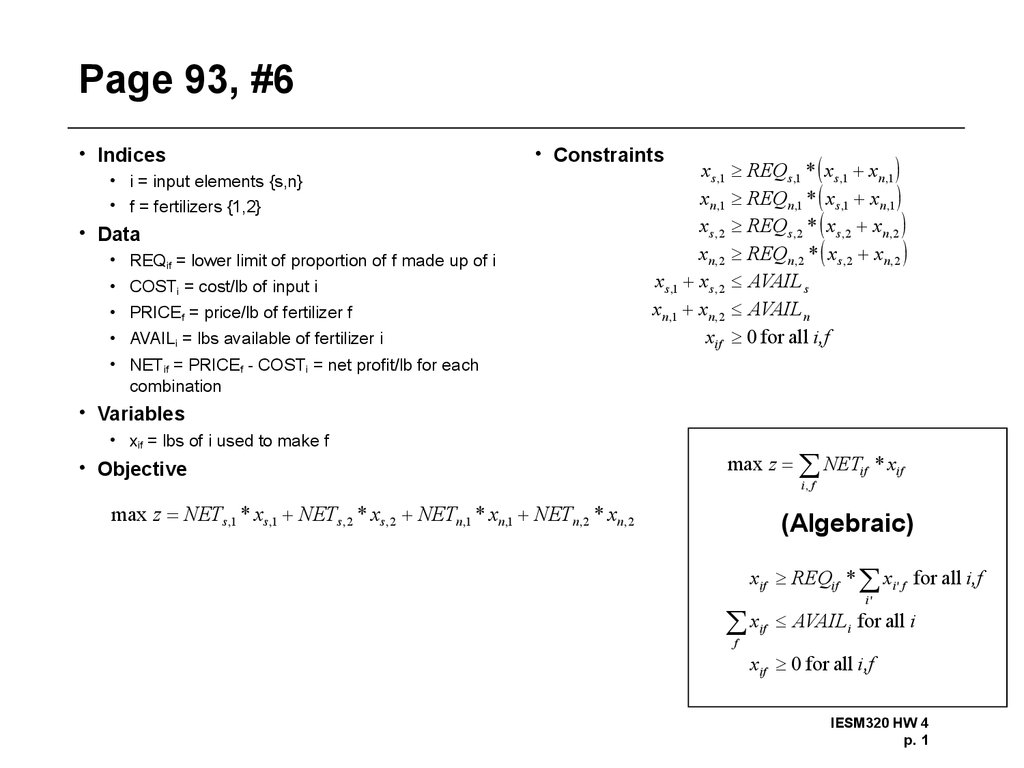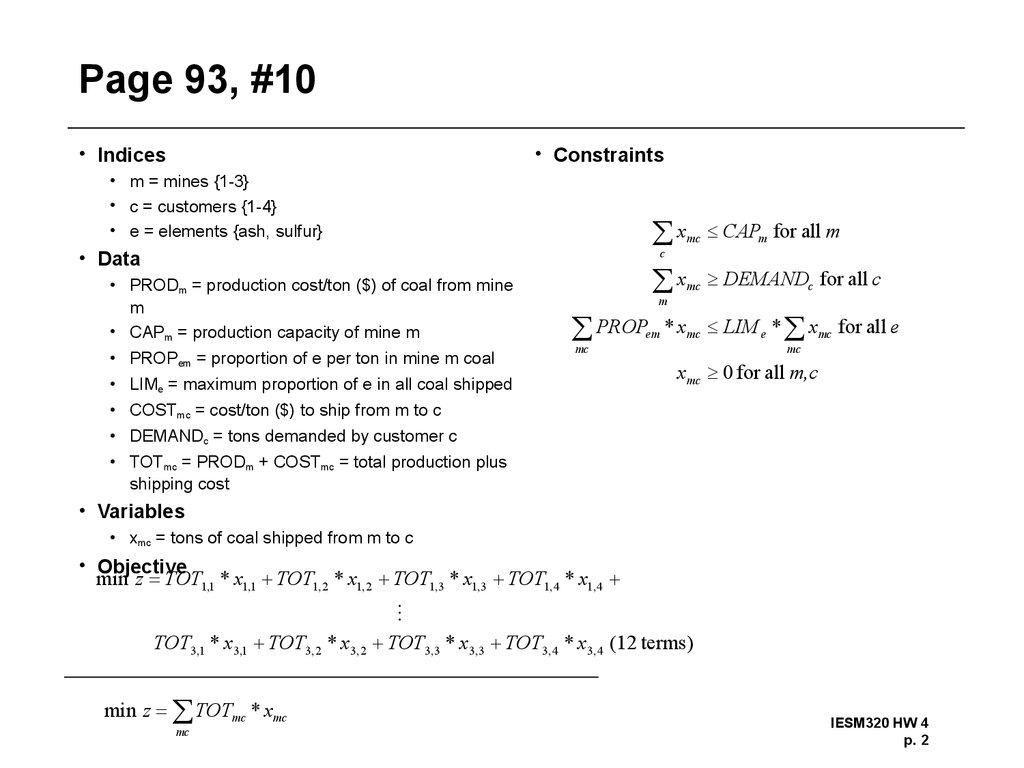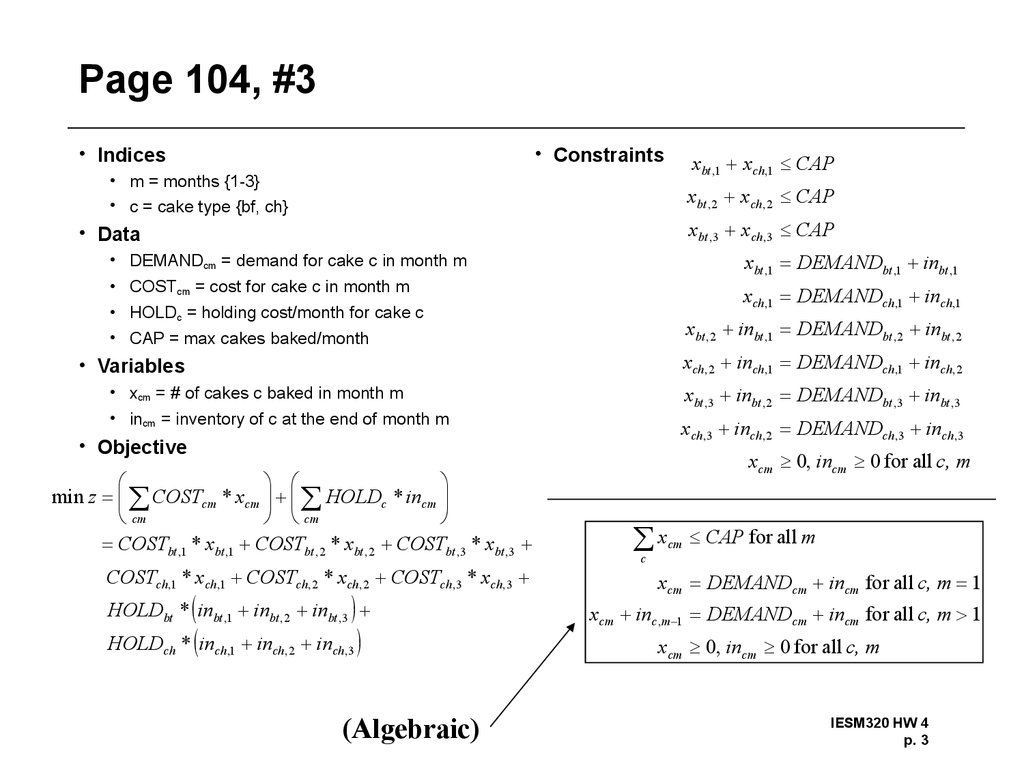# Homework #4 Solutions

## 1. Page 93, #6

• Indices
• Constraints
• i = input elements {s,n}
• f = fertilizers {1,2}
• Data
• REQif = lower limit of proportion of f made up of i
• COSTi = cost/lb of input i
• PRICEf = price/lb of fertilizer f
• AVAILi = lbs available of fertilizer i
x s ,1 REQs ,1 * xs ,1 xn ,1
xn ,1 REQn ,1 * x s ,1 xn ,1
x s ,2 REQs ,2 * x s ,2 xn ,2
xn ,2 REQn ,2 * x s ,2 xn ,2
xs ,1 x s ,2 AVAIL s
xn ,1 xn ,2 AVAIL n
xif 0 for all i,f
• NETif = PRICEf - COSTi = net profit/lb for each
combination
• Variables
• xif = lbs of i used to make f
• Objective
max z NETif * xif
i, f
max z NETs ,1 * xs ,1 NETs , 2 * xs , 2 NETn ,1 * xn ,1 NETn , 2 * xn , 2
(Algebraic)
xif REQif * xi ' f for all i,f
x
i'
if
AVAIL i for all i
f
xif 0 for all i,f
IESM320 HW 4
p. 1

## 2. Page 93, #10

• Indices
• Constraints
• m = mines {1-3}
• c = customers {1-4}
• e = elements {ash, sulfur}
x
mc
CAPm for all m
x
mc
DEMANDc for all c
c
• Data
• PRODm = production cost/ton (\$) of coal from mine
m
• CAPm = production capacity of mine m
• PROPem = proportion of e per ton in mine m coal
PROP
m
em
mc
• LIMe = maximum proportion of e in all coal shipped
• COSTmc = cost/ton (\$) to ship from m to c
* xmc LIM e * xmc for all e
mc
xmc 0 for all m,c
• DEMANDc = tons demanded by customer c
• TOTmc = PRODm + COSTmc = total production plus
shipping cost
• Variables
• xmc = tons of coal shipped from m to c
• Objective
min z TOT1,1 * x1,1 TOT1, 2 * x1, 2 TOT1,3 * x1,3 TOT1, 4 * x1, 4
TOT3,1 * x3,1 TOT3, 2 * x3, 2
min z TOTmc * xmc
mc
TOT3,3 * x3,3 TOT3, 4 * x3, 4 (12 terms)
IESM320 HW 4
p. 2

## 3. Page 104, #3

• Indices
• Constraints
xbt ,1 xch,1 CAP
• m = months {1-3}
• c = cake type {bf, ch}
xbt , 2 xch, 2 CAP
xbt ,3 xch ,3 CAP
• Data
xbt ,1 DEMANDbt ,1 inbt ,1
• DEMANDcm = demand for cake c in month m
• COSTcm = cost for cake c in month m
xch ,1 DEMANDch ,1 inch ,1
• HOLDc = holding cost/month for cake c
• CAP = max cakes baked/month
xbt , 2 inbt ,1 DEMANDbt , 2 inbt , 2
xch, 2 inch ,1 DEMANDch ,1 inch , 2
• Variables
xbt ,3 inbt , 2 DEMANDbt ,3 inbt ,3
• xcm = # of cakes c baked in month m
• incm = inventory of c at the end of month m
xch ,3 inch, 2 DEMANDch ,3 inch ,3
• Objective
min z COSTcm * xcm HOLDc * incm
cm
cm
COSTbt ,1 * xbt ,1 COSTbt , 2 * xbt , 2 COSTbt ,3 * xbt ,3
COSTch,1 * xch,1 COSTch , 2 * xch , 2 COSTch ,3 * xch,3
HOLDbt * inbt ,1 inbt , 2 inbt ,3
HOLDch * inch ,1 inch , 2 inch,3
(Algebraic)
xcm 0, incm 0 for all c, m
x
cm
CAP for all m
c
xcm DEMANDcm incm for all c, m 1
xcm inc ,m 1 DEMANDcm incm for all c, m 1
xcm 0, incm 0 for all c, m
IESM320 HW 4
p. 3

## 4. Page 104, #4

• Indices
• Constraints
• p = products {A,B}
• a = assembly lines {1,2}
• m = month {mar,apr}
x pam
PRODRATE
p
• Data
INIT p x pam DEMAND pm in pm for all p, m " mar"
• DEMANDpm = demand for p in m
• HOURSam = line hours of a available in m
x
PRODRATEpa = units of p produced/hour on a
PRODCOST = \$/hour to run a line
CARRY = carrying cost (\$)/unit/month
INITp = initial inventory of p
a
pam
in p ,m 1 DEMAND pm END p for all p, m " apr"
a
x pam 0 for all p, a, m
in pm 0 for all p, m
• ENDp = ending inventory of p
• Variables
• xpam = number of p produced on a in m
• inpm = ending inventory of p in month m
• Objective
x pam
min z PRODCOST *
pam PRODRATE pa
HOLD * in pm
pm
HOURS am for all a, m
pa
Note: problem defines
PRODRATE as hours/product,
which is strange. I divide here
because a rate is normally
products/hour; if you use the
data as given, you’d multiply
IESM320 HW 4
p. 4